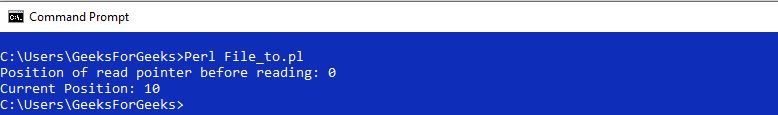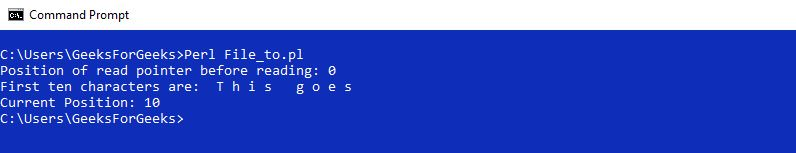Open in App
Not now

# Perl | tell() Function

• Last Updated : 07 May, 2019

tell() function in Perl is used to get the position of the read pointer in a File with the use of its FileHandle. If no FileHandle is passed then it returns the position within the most recent accessed file.

Syntax: tell(FileHandle)

Parameter:
FileHandle: Filehandle of the file to be accessed.

Returns: the current position of the read pointer

Example 1:

 `#!/usr/bin/perl  ` `  `  `# Opening a File in Read-only mode  ` `open``(fh, ``"<"``, ``"Hello.txt"``);  ` ` `  `\$position` `= ``tell``(fh); ` `print``(``"Position of read pointer before reading: \$position"``); ` `  `  `# Reading first 10 characters from the file ` `for``(``\$i` `= 0; ``\$i` `< 10; ``\$i``++) ` `{ ` `    ``\$ch` `= ``getc``(fh); ` `} ` ` `  `\$position` `= ``tell``(fh); ` ` `  `# Current position of the read pointer ` `print``(``"\nCurrent Position: \$position"``); ` `  `  `# Closing the File  ` `close``(fh);  `

Output:Example 2:

 `#!/usr/bin/perl  ` `  `  `# Opening a File in Read-only mode  ` `open``(fh, ``"<"``, ``"Hello.txt"``);  ` ` `  `\$position` `= ``tell``(fh); ` `print``(``"Position of read pointer before reading: \$position\n"``); ` ` `  `# Printing First 10 Characters ` `print``(``"First ten characters are: "``); ` ` `  `# Reading and printing first  ` `# 10 characters from the file ` `for``(``\$i` `= 0; ``\$i` `< 10; ``\$i``++) ` `{ ` `    ``\$ch` `= ``getc``(fh); ` `    ``print``" \$ch"``; ` `} ` ` `  `\$position` `= ``tell``(fh); ` ` `  `# Current position of the read pointer ` `print``(``"\nCurrent Position: \$position"``); ` `  `  `# Closing the File  ` `close``(fh);  `

Output:My Personal Notes arrow_drop_up
Related Articles